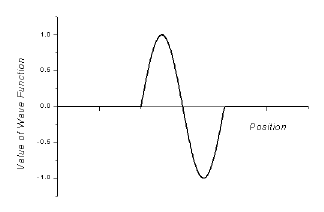Exploring Probabilities

When we square the wave function, we obtain the probability density. This graph is related to the probability of finding the electron at each point in space. However, we measure over regions in space. Fortunately, a little math can convert a probability density in a region into a number representing the probability of finding the object in that region. All of this is likely to seem somewhat abstract. We can make it more concrete with the Probability Illustrator program.

Click and drag the pencil that appears in the top frame to “sketch” the wave function similar to the one in the figure below. You need not sketch the wave function accurately. Anything that looks similar is acceptable for our purpose. The program sketches a graph of the probability density in the lower frame.

Does the probability density graph drawn by the program seem to be the square of the wave function that you sketched?

Now do the same for a wave function that is an approximate reflection (about the x-axis) of the previous one. (Something like the sketch below.)How do the probability density graphs in the two cases, compare with each other?

Is this result what you would expect? Why or why not?